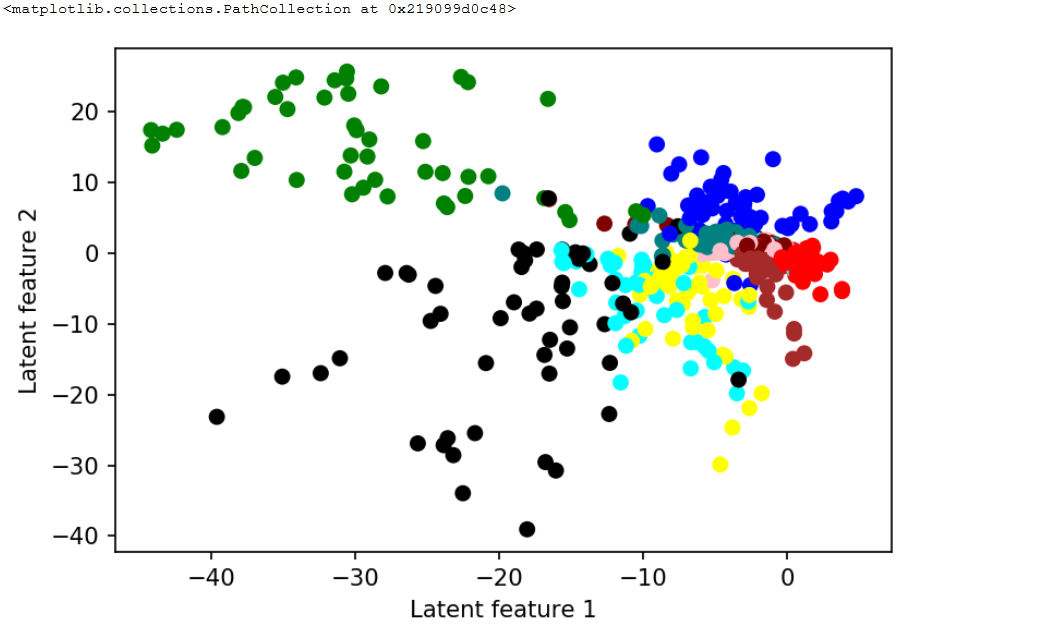# PyTorch | Autoencoder Example

## What are Autoencoders

Autoencoders are neural nets that do Identity function: $f(X) = X$.

The simplest Autoencoder would be a two layer net with just one hidden layer, but in here we will use eight linear layers Autoencoder.

Autoencoder has three parts:

• an encoding function,
• a decoding function, and
• a loss function

The encoder learns to represent the input as latent features. The decoder learns to reconstruct the latent features back to the original data.

## Create Autoencoder using MNIST

In here I will create and train the Autoencoder with just two latent features and I will use the features to scatter plot an interesting picture. I am using the MNIST dataset.

import torch
import torchvision
from torch import nn
from torch.utils.data import DataLoader
from torchvision import transforms
from torchvision.datasets import MNIST

img_transform = transforms.Compose([
transforms.ToTensor(),
transforms.Normalize([0.5], [0.5])
])

dataset = MNIST('./data', transform=img_transform, download=True)

class LAutoencoder(nn.Module):
def __init__(self):
super().__init__()
self.encoder = nn.Sequential(
nn.Linear(28 * 28, 128),
nn.ReLU(True),
nn.Linear(128, 64),
nn.ReLU(True),
nn.Linear(64, 12),
nn.ReLU(True),
nn.Linear(12, 2))
self.decoder = nn.Sequential(
nn.Linear(2, 12),
nn.ReLU(True),
nn.Linear(12, 64),
nn.ReLU(True),
nn.Linear(64, 128),
nn.ReLU(True),
nn.Linear(128, 28 * 28),
nn.Tanh() )

def forward(self, x):
latent = self.encoder(x)
x = self.decoder(latent)
return x,latent


Out:

Using downloaded and verified file: ./data\MNIST\raw\train-images-idx3-ubyte.gz
Extracting ./data\MNIST\raw\train-images-idx3-ubyte.gz to ./data\MNIST\raw
Downloading http://yann.lecun.com/exdb/mnist/train-labels-idx1-ubyte.gz to ./data\MNIST\raw\train-labels-idx1-ubyte.gz

HBox(children=(IntProgress(value=1, bar_style='info', max=1), HTML(value='')))

Extracting ./data\MNIST\raw\train-labels-idx1-ubyte.gz to ./data\MNIST\raw
Downloading http://yann.lecun.com/exdb/mnist/t10k-images-idx3-ubyte.gz to ./data\MNIST\raw\t10k-images-idx3-ubyte.gz

HBox(children=(IntProgress(value=1, bar_style='info', max=1), HTML(value='')))

Extracting ./data\MNIST\raw\t10k-images-idx3-ubyte.gz to ./data\MNIST\raw
Downloading http://yann.lecun.com/exdb/mnist/t10k-labels-idx1-ubyte.gz to ./data\MNIST\raw\t10k-labels-idx1-ubyte.gz

HBox(children=(IntProgress(value=1, bar_style='info', max=1), HTML(value='')))

Extracting ./data\MNIST\raw\t10k-labels-idx1-ubyte.gz to ./data\MNIST\raw
Processing...
Done!


## Training

num_epochs = 10
batch_size = 128
learning_rate = 2e-3
dataloader = DataLoader(dataset, batch_size=batch_size, shuffle=True)

model = LAutoencoder()
model.cuda()
criterion = nn.MSELoss()
optimizer = torch.optim.AdamW(
model.parameters(), lr=learning_rate)

for epoch in range(num_epochs):
for data in dataloader:
img, labels = data
img = img.view(img.size(0), -1).cuda()

output,latent = model(img)
loss = criterion(output, img)

loss.backward()
optimizer.step()
optimizer.zero_grad()

print(f'epoch [{epoch + 1}/{num_epochs}], loss:{loss.data.item()}')


Out:

epoch [1/10], loss:0.18733486533164978
epoch [2/10], loss:0.18520425260066986
epoch [3/10], loss:0.16901877522468567
epoch [4/10], loss:0.15758267045021057
epoch [5/10], loss:0.156107559800148
epoch [6/10], loss:0.16009172797203064
epoch [7/10], loss:0.158538356423378
epoch [8/10], loss:0.16316969692707062
epoch [9/10], loss:0.16268609464168549
epoch [10/10], loss:0.15088655054569244


## The results

%matplotlib inline
import matplotlib
import matplotlib.pyplot as plt
import numpy as np

dataloader = DataLoader(dataset, batch_size=512, shuffle=True)
for data in dataloader:
img, labels = data
img = img.view(img.size(0), -1)
model.cpu()
_,latent = model(img)
break

d = {0: 'red', 1: "green", 2: "blue", 3: "maroon", 4: "yellow",
5: "pink", 6: "brown", 7: "black", 8: "teal", 9: "aqua"}

colors = []
for e in labels.numpy():
colors.append(d[e])

fig = plt.figure(dpi=153)
ax = fig.add_subplot(111)
ax.set_xlabel('Latent feature 1')
ax.set_ylabel('Latent feature 2')

ax.scatter(latent[:,0].detach().numpy(), latent[:,1].detach().numpy(),
c=list(colors))Note with more latent features we can get better separation

You may note LAutoencoder has exactly 2 latent features between the encoder and the decoder. Encoder ends with the nn.Linear(12, 2)), and the decoder starts with the nn.Linear(2, 12). To create a scatter plot we first grab images and labels.

Single batch of images was 512. Then we calculated the latent features for all the batch images together with the labels from 0 to 9.

latent[:,0].detach().numpy() is for the first feature, and latent[:,1].detach().numpy() for the second feature. We mapped each label from 0 to 9 to colors. In the end we got the landscape of points and we may understand the colors are grouped.

If we increase of number of latent features it becomes easier to isolate points of same color.

To create the convolutional Autoencoder we woudl use nn.Conv2d together with the nn.ConvTranspose2d modules.

class CAutoencoder(nn.Module):

def __init__(self):
super(Autoencoder,self).__init__()

self.encoder = nn.Sequential(
nn.Conv2d(1, 6, kernel_size=5),
nn.ReLU(True),
nn.Conv2d(6,16,kernel_size=5),
nn.ReLU(True))

self.decoder = nn.Sequential(
nn.ConvTranspose2d(16,6,kernel_size=5),
nn.ReLU(True),
nn.ConvTranspose2d(6,3,kernel_size=5),
nn.ReLU(True))

def forward(self,x):
x = self.encoder(x)
x = self.decoder(x)
return x


There is a special type of Autoencoders called Variational Autoencoders (VAE), appeared in the work of Diederik P Kingma and Max Welling.

One very useful usage of VAE may be image denoising.

tags: project - autoencoder - tutor & category: pytorch